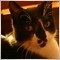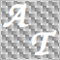• Übersicht
• Bewertungen (3)
• Diskussion (15)
• Neue Funktionen

Volatility Predictor

The indicator predicts the future volatility with a probability close to 2/3. There are two operation modes:

1. In the first mode, the chart displays the probable future values of the prices.
2. In the second mode, the chart displays the estimated values, indicating either the levels that may be broken with a probability of 2/3, or the boundaries of the range, within which the price is expected to stay with the same probability.

The prediction is based on the dependence of the future value on the volatility ratio of the last period and the one before it. If the current volatility decreases compared to the previous one, then the future volatility will be greater than the current one with probability of about 66%, and less than the previous one with probability of about 66%.

In case when the current volatility increases, the reverse regularity is valid. Thus, by comparing the values of the history volatility, it is possible to predict the future volatility with high probability, using the long-observed property of so-called volatility cyclicity, which, despite the sufficient popularity, still has not been implemented as predicting indicators, which would be included in the standard sets of the trading terminals.

By predicting the volatility, it is possible to determine good points for placing stop loss and take profit levels, and also to filter the position entry signals. In addition, it is possible to trade the volatility directly using the option contracts.

Indicator parameters

• period - volatility calculation period.
• indicator_mode - selection of the indicator operation mode.4270
2018.07.12 21:25

Der Benutzer hat keinen Kommentar hinterlassen336
2018.04.17 15:31

Works on all time frames and pairs and is surprisingly accurate.21262
2016.08.31 08:10

Der Benutzer hat keinen Kommentar hinterlassen

Version 2.0 2019.06.09
New function - history bars amount to count and draw indicator lines.